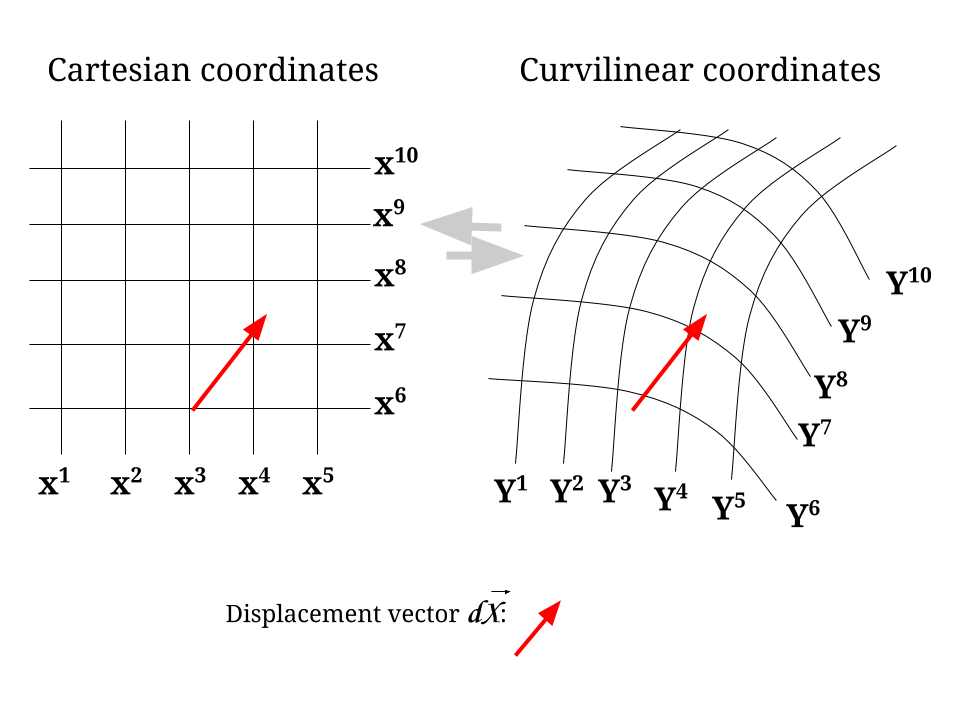### The Metric Tensor:The length or magnitude of the vector $$dS$$ in Cartesian coordinates is given by:

$dS^2=\left(dX^1\right)^2 + \left(dX^2\right)^2 + \left(dX^3\right)^2 + \cdots = \large \delta_{mn} \;\color{blue}{dX^m}\, dX^n\tag 1$

Notice that the dummy indexes are set up to sum over. We start with $$m=1$$, and we see that all terms are going to be zero unless when $$n=1$$, in which case it will be $$dX^1\, dX^1 = (dX^1)^2.$$

But how to write the same for the curvilinear system?

We have to refer back to the following expression of a change of coordinate system at the beginning of the notes on tensors:

$dy^n = \frac{\partial y^n}{\partial x^\color{blue}{m}} dx^{\color{blue}{m}} \tag{Ref.1}$

In this case we have that the part in blue in Eq. 1 can be expressed as:

$\color{blue}{dX^m}= \frac{\partial x^m}{\partial y^r} dy^r \tag2$

Hence, Eq. 1 becomes,

$dS^2 = \large \delta_{mn} \;dX^m\, dX^n= \large \underset{\text{METRIC TENSOR }\Large g^{(y)}_{\color{red}{rs}}}{\underbrace{\delta_{mn}\frac{\partial x^m}{\partial y^\color{red}{r}}\frac{\partial x^n}{\partial y^\color{red}{s}}}} \;dy^r\; dy^s$

$\Large \bbox[10px, border:2px solid aqua]{g^{(y)}_{\color{red}{rs}}=\delta_{mn}\frac{\partial x^m}{\partial y^\color{red}{r}}\frac{\partial x^n}{\partial y^\color{red}{s}}}\tag 3$

Here is another derivation: